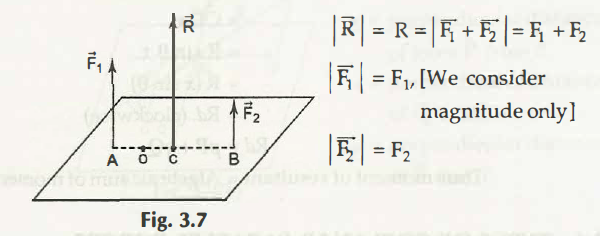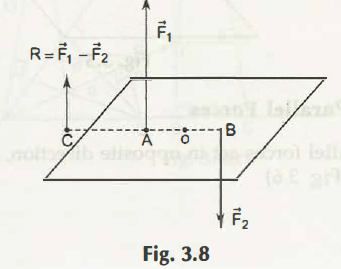3.5.1 Resultant of Two Like Coplanar Parallel Forces –

F1 and F2 are the two similar parallelforces that result in R = F1 + F2. Therefore we apply Varignon’s principle, and the following is obtained:Or                    -F1 x AO +F2 x BO =R X CO =(F1+F2) CO

Or                    -F1 X AO +F2 X BO =F1 X CO +F2 X CO

Or                                   F1 X (AO+CO) = F 2  (BO-CO)

Or                                                        F1 X AC =F2 X BC

Alternatively,                                                 F1/F2 =BC/AC.

Thus the conclusion is that C is the point the resultant point where the force acts.

3.5.2 Resultant of Two Coplanar Unlike Parallel Forces

Hence we relate the principle of moments to the equation given below:

O- F l X AO – F 2 X BO = -R X CO= -R X CO

= – (F1-F2) CO

-F1 X AO –F2 X BO = – F1 XCO +CO +F2 X CO

F1 (CO –AO)

=F2 (BO+CO)

F1 X AC =F= X BC

F1 /F2 =BC/AC .

Thus the point C which is the fixed point of force application.

Links of Next Mechanical Engineering Topics:-### Customer Reviews

My Homework Help
Rated 5.0 out of 5 based on 510 customer reviews at